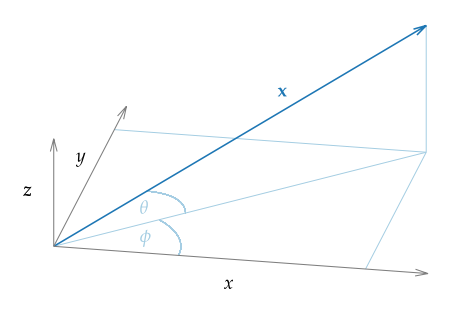# 2. Mathematical Definitions¶

## 2.1. Coordinate system¶

Fig. 2.1 shows the coordinate system that is used in the following chapters. A vector $$\x$$ can be described by its position $$(x,y,z)$$ in space or by its length, azimuth angle $$\phi \in [0,2\pi[$$, and elevation $$\theta \in \left[-\frac{\pi}{2},\frac{\pi}{2}\right]$$. The azimuth is measured counterclockwise and elevation is positive for positive $$z$$-values.Fig. 2.1 Coordinate system used in this document. The vector $$x$$ can also be described by its length, its azimuth angle $$\phi$$, and its elevation $$\theta$$.

## 2.2. Fourier transformation¶

Let $$s$$ be an absolute integrable function, $$t,\w$$ real numbers, then the temporal Fourier transform is defined after [Bra00] as

(2.1)$S(\w) = \mathcal{F}\left\{s(t)\right\} = \int^{\infty}_{-\infty} s(t) \e{-\i\w t} \d t.$

In the same way the inverse temporal Fourier transform is defined as

(2.2)$s(t) = \mathcal{F}^{-1}\left\{S(\w)\right\} = \frac{1}{2\pi} \int^{\infty}_{-\infty} S(\w) \e{\i\w t} \d\w.$